Search IntMath
Close

# Factorials and the Gamma function

By Murray Bourne, 14 Apr 2010

In math, we often come across the following expression:

n!

This is "n factorial", or the product

n(n − 1)(n − 2)(n − 3) ... (3)(2)(1).

Factorials are used in the study of counting and probability. For example, permutations (which involves counting the arrangement of objects where the order is important) and combinations (where the order is not important) both require factorials when the number of objects is large.Also, finding the probability of winning Lotto or cards also involves factorials.

Examples of factorials:

2! = 2 × 1 = 2

3! = 3 × 2 × 1 = 6

4! = 4 × 3 × 2 × 1 = 24

5! = 5 × 4 × 3 × 2 × 1 = 120## Product Notation

We can write factorials using product notation (upper case "pi") as follows: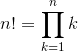This notation works in a similar way to summation notation (Σ), but in this case we multiply rather than add terms. For example, if n = 4, we would substitute k = 1, then k = 2, then k = 3 and finally k = 4 and write: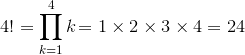0! is a special case. There are many situations where the value of 0! has a meaning, and it has value 1 in those cases, not 0. So by convention, we define:

0! = 1

Also, 1! has value 1:

1! = 1

The graph of f(n) = n! for integer values n is as follows:Note that points A(0,1), B(1,1), C(2,2), D(3,6), E(4,24), and F(5, 120) are discrete points in space - we have not connected them with a curve. There is no meaning for non-integer factorials like the expression 3.5! (3.5 factorial).

However, our graph does suggest a curve - one that is (approximately) exponentially increasing.

Is there a function we can use that fits this curve and so gives us meaningful values for factorials of numbers which are not whole numbers?

It turns out there is.

## The Gamma Function

The Gamma Function is an extension of the concept of factorial numbers. We can input (almost) any real or complex number into the Gamma function and find its value. Such values will be related to factorial values.

There is a special case where we can see the connection to factorial numbers.

If n is a positive integer, then the function Gamma (named after the Greek letter "Γ" by the mathematician Legendre) of n is:

Γ(n) = (n − 1)!

We can easily "shift" this by 1 and obtain an expression for n! as follows:

Γ(n + 1) = n!

But the Gamma function is not restricted to the whole numbers (that's the point). A formula that allows us to find the value of the Gamma function for any real value of n is as follows: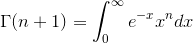For example, let n = 3.5. We want to find the value of 3.5!, assuming it exists.

The value of Γ(3.5 + 1) = Γ(4.5) is given by the infinite integral: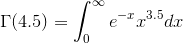Examining the graph above, we expect this value to be somewhere in the range 10 to 15. (Recall that 3! = 6 and 4! = 24. Our answer for Γ(4.5) = 3.5! has to be between these values.)

## What does this integral mean?

The function under the integral sign is very interesting. It is the product of an ever-decreasing function with an ever-increasing one.

f(x) = exx3.5

Let's look at the graphs involved in this expression.

Firstly, f(x) = ex. Note that the value of the function (its height) decreases as x increases.Secondly, f(x) = x3.5 increases as x increases. This function is not defined (over the reals) for negative x.Finally, we look at the product of the 2 functions. This is the graph of f(x) = exx3.5.The area under this graph (the shaded portion) from 0 to ∞ (infinity) gives us the value of Γ(4.5) = 3.5!.

We use computer mathematics software (Scientific Notebook) to find the value of the integral. We only need to choose some "large number" (I chose 10000) for the upper bound of the integral since as you can see in the graph, the height of the curve is very small as x becomes very large. Here's what we get: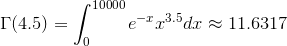This means the shaded area above is 11.6317 square units. This is in the range we estimated earlier.

## Back to the Graph

Let's return to our graph of the values of factorial numbers. We can use the above integral to calculate values of the Gamma function for any real value of n.

This time, I have included a smooth curve passing through our factorial values. This curve is f(n) = Γ(n + 1).

I've also added the new point F(3.5, 11.6317), which is the ordered pair representing Γ(4.5) = 11.6317 we just found. You can see this new point lies on the smooth curve joining the other factorial values.## Conclusion

The Gamma function gives us values that are analogous to factorials of non-integer numbers. It was one of the many brilliant contributions to the world of math by the Swiss mathematician Leonhard Euler.

To finish, let's look at the graph of f(n) = Γ(n + 1) for a greater range of real values of n. We observe there are some "holes" (discontinuities) in the graph for the negative integers.### Comment Preview

HTML: You can use simple tags like <b>, <a href="...">, etc.

To enter math, you can can either:

1. Use simple calculator-like input in the following format (surround your math in backticks, or qq on tablet or phone):
a^2 = sqrt(b^2 + c^2)
(See more on ASCIIMath syntax); or
2. Use simple LaTeX in the following format. Surround your math with $$ and $$.
$$\int g dx = \sqrt{\frac{a}{b}}$$
(This is standard simple LaTeX.)

NOTE: You can mix both types of math entry in your comment.